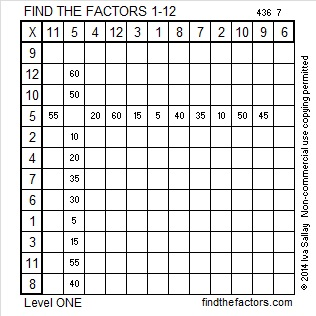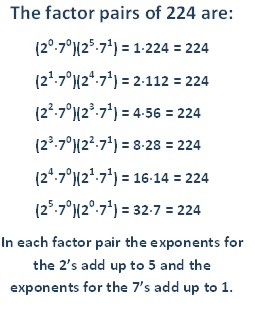# 226 and Level 1

• 226 is a composite number.
• Prime factorization: 226 = 2 x 113
• The exponents in the prime factorization are 1, and 1. Adding one to each and multiplying we get (1 + 1)(1 + 1) = 2 x 2 = 4. Therefore 226 has 4 factors.
• Factors of 226: 1, 2, 113, 226
• Factor pairs: 226 = 1 x 226 or 2 x 113
• 226 has no square factors so its square root cannot be simplified. √226 ≈ 15.033Excel file of puzzles and previous week’s factor solutions: 12 Factors 2014-09-08# How Many Factors Does 224 Have, and Why Does It Have That Many?

• 224 is a composite number.
• Prime factorization: 224 = 2 x 2 x 2 x 2 x 2 x 7, which can be written 224 = 2⁵ x 7
• The exponents in the prime factorization are 5 and 1. Adding one to each and multiplying we get (5 + 1)(1 + 1) = 6 x 2 = 12. Therefore 224 has 12 factors.
• Factors of 224: 1, 2, 4, 7, 8, 14, 16, 28, 32, 56, 112, 224
• Factor pairs: 224 = 1 x 224, 2 x 112, 4 x 56, 7 x 32, 8 x 28, or 14 x 16
• Taking the factor pair with the largest square number factor, we get √224 = (√16)( √14) = 4√14 ≈ 14.967It may seem like a little mathemagic to state that 224 has 12 factors because the exponents in its prime factorization are 5 and 1 and because 6 x 2 = 12. If we look at the factors of 224 in a different way, all of the mathemagician’s secrets will be revealed.

First it must be understood that any number (except zero) raised to the zeroth power is equal to one.

Since the prime factorization of 224 is 2⁵ x 7¹, every one of its factors can be written as the product of certain powers of 2 and 7:Counting all the factors of 224 is similar to counting the number of possible sandwiches when there are 5 different meats (and vegetarians are given the option of no meat) and one type of bread (and those counting carbs are given the option of no bread). Even though there are 5 different types of meat, there are 6 possible choices about meat, while bread or no bread makes 2 possible choices about bread. The fundamental counting principle states that we can count the total number of possible sandwiches by multiplying together the number of possible choices of meat and bread. In this case that is 6 x 2 = 12.

(We are allowing people to chose to have no meat and/or no bread. Usually when the fundamental counting principle is used we don’t make that allowance.  Allowing the sandwiches to be made without meat and/or bread means we have to add 1 to the 5 types of meat and 1 to the 1 type of bread before we multiply those numbers together, giving us (5 + 1)(1 + 1) or 6 x 2. If we did not allow anyone to choose to leave out the meat and/or bread we would not add 1.)

Adding even one more number in the prime factorization or adding even one more ingredient to the sandwich can make counting the factors or sandwiches more tedious:

If we also allow 3 different types of lettuce (or no lettuce for those who don’t want any), the problem becomes a little more complicated, but the fundamental counting principle makes it easy to find the number of possible types of sandwiches. Since we now have 4 lettuce choices, we simply multiply the previous information by that number: (6 x 2) x 4 = 12 x 4 = 48. Finding the number of possible sandwiches is easy, but listing all 48 sandwiches might make some people lose their appetite.

Just as it worked for finding the number of possible sandwiches, the fundamental counting principle also helps us know the number of factors a whole number has. When each number in a factor pair is written using a modified version of its prime factorization (to allow us to write a factor to the zeroth power), then we can clearly see how to make (2^5) x (7 ^1) with every factor pair.If the prime factorization of a number were (2^5) x (7^1) x (11^3), it would have (5+1)(1+1)(3+1) = 6 x 2 x 4 = 48 factors. Finding the number of factors may be much easier than listing all of them, but knowing how many factors there are helps us make sure we don’t list too few or too many.

When a number is prime such as 7, it has no prime factorization, but 7 can be represented as (7^1). Its exponent is 1; (1 + 1) = 2, and its two factors can be listed as (7^0) and (7^1) or 1 and 7. All prime numbers have exactly 2 factors.

Factor pairs obviously come in 2’s, so will the number of factors a number has always be an even number?

We know from number theory when we multiply an even number by any other number, we always get an even number. To get an odd number as the product, EVERY number that is multiplied together would have to be odd. Therefore, the only way to get an odd number when we use the fundamental counting principle is if all the numbers being multiplied together are odd. In the case of counting the number of factors of a number, we only get an odd number of factors when the number being factored is a perfect square. That is the only way ALL of the exponents in its prime factorization are even numbers, and those even exponents plus 1 will ALL be odd.

For example, if the prime factorization were (2^6)(3^2), then the number of factors would be (6 + 1)(2 + 1) = 7 x 3 = 21, a odd number. One of its factor pairs could be written (2^3)(3^1) x (2^3)(3^1), but is normally listed as 24 x 24. So when we list the factor pairs there will be 11 pairs, but when we list the 21 factors of 576, we only list 24 once. Fortunately teachers and textbooks rarely choose numbers with that many factors.

When factoring numbers, making sandwiches, or making many other choices, the fundamental counting principle gives the number of all the possible outcomes and makes it easier not to overlook any possibilities.